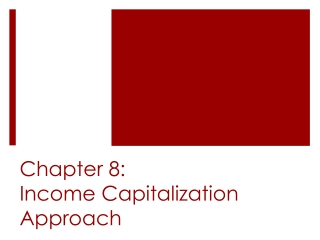DownloadDownload PresentationChapter 8: Income Capitalization Approach

# Chapter 8: Income Capitalization Approach

Télécharger la présentation## Chapter 8: Income Capitalization Approach

- - - - - - - - - - - - - - - - - - - - - - - - - - - E N D - - - - - - - - - - - - - - - - - - - - - - - - - - -
##### Presentation Transcript

1. Chapter 8:Income Capitalization Approach

2. The Income Approach to Valuation Value determined as present value of future benefits Perspective of typical investor

3. Income Capitalization Methods • Direct Capitalization • Converts a single year’s income into an indication of value using an income rate or income multiplier. • Advantage is that cap rates and income multipliers come directly from market indications. • Disadvantage is that expected changes in income will be similar for the comparable properties and the subject property. • Yield Capitalization • Converts expected future cash flows into an indication of value using discounted cash flow analysis. • Advantage is that the income each year is explicitly identified.

4. Application of the Income Approach • Office buildings • Expense passthrough • Consumer price index (CPI) • Consumer price index (CPI) • Leased fee interest • Retail shopping centers • Minimum Rent • Overage Rent • Percentage Rent

5. Application of the Income Approach • Multifamily residential properties • Industrial properties • Hotels and Motels • Nonrealty • Business Value • Development Projects • Special-Use Properties

6. Value Estimation Using Direct Capitalization • Appropriate when: • First-year cash flows are representative of an average or normal year’s earnings • The income multiplier or capitalization rate is derived from comparable sales with the same potential for future income.

7. Potential Gross Income Multiplier • First, the PGIM is obtained by extracting PGIMs from recent sales of similar properties in the area and calculating the average PGIM: • PGIM=Value/PGI • Then, use that PGIM to calculate the value of your subject property using the following equation: • Value of subject=PGI of subject x PGIM

8. Effective Gross Income Multiplier • More appropriate to use when long-term differences in vacancy between subject and comparable occur. • First, the EGIM is obtained by extracting EGIMs from recent sales of similar properties in the area and calculating the average EGIM: • EGIM=Value/EGI • Then, use that EGIM to calculate the value of your subject property using the following equation: • Value of subject=EGI of subject x EGIM

9. Net Income Multiplier • More appropriate to use when long-term differences in expense ratios between subject and comparable occur. • First, the NIM is obtained by extracting NIMs from recent sales of similar properties in the area and calculating the average NIM: • NIM=Value/NOI • Then, use that NIM to calculate the value of your subject property using the following equation: • Value of subject=NOI of subject x NIM

10. Capitalization Rate • We can also use Net Income as a tool for estimating valuation with a capitalization rate. • First, the cap rate is obtained by extracting cap rates from recent sales of similar properties in the area and calculating the average cape rate: • Cap Rate=NOI/Value • Then, use that Cap Rate to calculate the value of your subject property using the following equation: • Value of subject=NOI of subject/Cap Rate

11. Residual Techniques Using Direct Capitalization: Building Residual Assuming the value of land is known but value of building is not, the value of components can be broken and total value estimated as follows:

12. Residual Techniques Using Direct Capitalization: Land Residual Assuming the value of the building is known but value of the land is not, the value of components can be broken and total value estimated as follows:

13. Residual Techniques Using Direct Capitalization: Land Residual The land residual technique was originally developed as a way to analyze highest and best use. The appraiser would create an estimate of land residual value for each development alternative. The alternative that yields the highest land residual value is considered the highest and best use. This approach, however, requires separate cap rate estimates for the land and building. This is difficult in practice, so an alternative is to use an overall cap rate calculation.

14. Value Estimation Using Yield Capitalization: Fixed Income and Resale Price If I=12%, solving for NPV gives a value estimate of \$2,026,037 Yield capitalization involves discounting unlevered cash flows at the property discount rate (target property yield for a given investor).

15. Value Estimation Using Yield Capitalization: Variable Income and Fixed Resale Price If I=12%, solving for NPV gives a value estimate of \$2,077,068

16. Value Estimation Using Yield Capitalization: Variable Income and Resale Price Based on Terminal Cap Rate Chapter 6 mentioned the topic of terminal cap rates, and this is where that concept gets used. The terminal cap rate can be applied to an estimate of income for the next owner to estimate the resale price. This income depends upon how market rates are expected to change over the first owner’s holding period and whether any of the original leases are still in effect.

17. Value Estimation Using Yield Capitalization: Variable Income and Resale Price Based on Terminal Cap Rate If Estimated NOI in year 6=\$241,480 and the Terminal cap rate=10% Resale value=Estimated NOI in year 6/Terminal cap rate Resale value = \$241,480/.10 = \$2,414,800

18. Value Estimation Using Yield Capitalization: Variable Income and Resale Price Based on Terminal Cap Rate Assuming a 5 year holding period, if I=12%, solving for NPV gives a value estimate of \$2,142,209